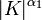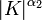# Difference between revisions of "Order of a profinite group need not determine order as a group in the sense of cardinality of underlying set"

It is possible to have two profinite groups$G_1$ and$G_2$ that have the same order as each other in the sense of order of a profinite group (note that both order numbers are supernatural numbers), but that have different orders from each other in the sense of the cardinality of the underlying set.
Fix a nontrivial finite group$K$. Pick two infinite cardinals$\alpha_1, \alpha_2$ such that the power cardinals of$\alpha_1,\alpha_2$ are not equal. Now, consider the external direct powers (repeated unrestricted external direct product of$K$ with itself):$G_1 = K^{\alpha_1}$ and$G_2 = K^{\alpha_2}$, both equipped with the product topology from the discrete topology on$K$. We note that:
• For both groups, the order in the sense of a profinite group is as follows: all primes that divide the order of$K$ occur with a power of$\infty$, but no other primes occur. In particular, the order of$G_1$ equals the order of$G_2$ in the sense of order as a profinite group, where the equality is as equality of supernatural numbers.
• The cardinality of the underlying set of$G_1$ is$|K|^{\alpha_1}$, which is the power cardinal of$\alpha_1$ (assuming the axiom of choice). The cardinality of the underlying set of$G_2$ is$|K|^{\alpha_2}$, which is the power cardinality of$\alpha_2$ (assuming the axiom of choice). By assumption, these two power cardinals are distinct.